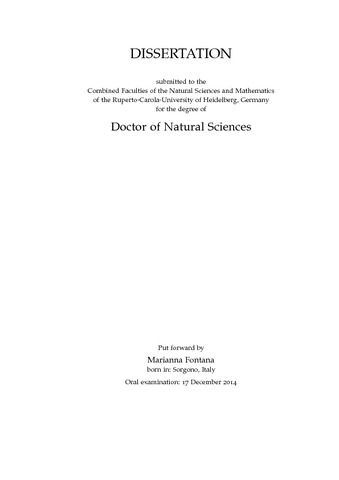## Search for the B_{d,s}^0 -> K^{*0} K_{S}^0 decays at LHCb

Fontana, MariannaPreview
PDF, English

Citation of documents: Please do not cite the URL that is displayed in your browser location input, instead use the DOI, URN or the persistent URL below, as we can guarantee their long-time accessibility.

## Abstract

The search for the previously unobserved \BdstoKstarKs decays is performed at the LHCb experiment, using proton-proton collisions at a centre of mass energy of $\sqrt{s}=7$ TeV. These decays provide a fertile ground in the search of New Physics in \CP violation measurements. The data sample was recorded in 2011 and corresponds to an integrated luminosity of 1 fb^{-1}. The first observation of the B_{s}^0 -> K^{*0} K_{S}^0 decay is reported. A measurement of the limit and the branching fraction relative to the B_{d}^0 -> K^{*0} K_{S}^0 decay yields the following results:

BF(B_{d}^0 -> K^{*0} K_{S}^0)/BF(B_{d,s}^0 -> K_{S}^0 \pi^+ \pi^-) < 0.12 (0.15) at 90\% (95\%) CL

BF(B_{s}^0 -> K^{*0} K_{S}^0)/BF(B_{d,s}^0 -> K_{S}^0 \pi^+ \pi^-) = 0.22 \pm 0.06(stat) \pm 0.02(syst) \pm 0.01(f_s/f_d),

where the first uncertainty is statistical, the second systematic and the third comes from the uncertainty on the hadronisation fraction.

Item Type: Dissertation Hofmann, Prof. Dr. Werner 17 December 2014 30 Jan 2015 10:56 2015 The Faculty of Physics and Astronomy > Institute of Physics 530 Physics